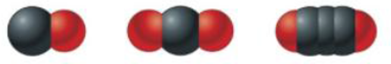# The three most stable oxides of carbon are carbon monoxide (CO), carbon dioxide (CO 2 ), and carbon suboxide (C 3 O 2 ). The space-filling models for these three compounds are For each oxide, draw the Lewis structure, predict the molecular structure, and describe the bonding (in terms of the hybrid orbitals for the carbon atoms).### Chemistry: An Atoms First Approach

2nd Edition
Steven S. Zumdahl + 1 other
Publisher: Cengage Learning
ISBN: 9781305079243

#### Solutions

Chapter
Section### Chemistry: An Atoms First Approach

2nd Edition
Steven S. Zumdahl + 1 other
Publisher: Cengage Learning
ISBN: 9781305079243
Chapter 4, Problem 90AE
Textbook Problem
1 views

## The three most stable oxides of carbon are carbon monoxide (CO), carbon dioxide (CO2), and carbon suboxide (C3O2). The space-filling models for these three compounds areFor each oxide, draw the Lewis structure, predict the molecular structure, and describe the bonding (in terms of the hybrid orbitals for the carbon atoms).

(I)

Interpretation Introduction

Interpretation: The Lewis structures and the molecular structures of the given compounds; and the bonding of these compounds are to be stated.

Concept introduction: The following steps are to be followed to determine the molecular structure of a given compound,

• The central atom is identified.
• Its valence electrons are determined.
• 1e is added to the total number of valence electrons for each monovalent atom present.
• The total number obtained is divided by 2 , to find the number of electron pairs.
• This further gives us the hybridization of the given compound.

To determine: The Lewis structure and the molecular structure of CO

### Explanation of Solution

The central atom in CO is carbon (C) . The electronic configuration of carbon is,

1s22s22p2

The valence electrons in carbon are six.

The electronic configuration of oxygen is,

1s22s22p4

The valence elctrons in oxygen is six.

The total number of valence electrons are,

C+O=(4+6)e=(10)e</

(II)

Interpretation Introduction

Interpretation: The Lewis structures and the molecular structures of the given compounds; and the bonding of these compounds are to be stated.

Concept introduction: The following steps are to be followed to determine the molecular structure of a given compound,

• The central atom is identified.
• Its valence electrons are determined.
• 1e is added to the total number of valence electrons for each monovalent atom present.
• The total number obtained is divided by 2 , to find the number of electron pairs.
• This further gives us the hybridization of the given compound.

To determine: The Lewis structure and the molecular structure of CO2

(III)

Interpretation Introduction

Interpretation: The Lewis structures and the molecular structures of the given compounds; and the bonding of these compounds are to be stated.

Concept introduction: The following steps are to be followed to determine the molecular structure of a given compound,

• The central atom is identified.
• Its valence electrons are determined.
• 1e is added to the total number of valence electrons for each monovalent atom present.
• The total number obtained is divided by 2 , to find the number of electron pairs.
• This further gives us the hybridization of the given compound.

To determine: The Lewis structure and the molecular structure of C3O2

### Still sussing out bartleby?

Check out a sample textbook solution.

See a sample solution

#### The Solution to Your Study Problems

Bartleby provides explanations to thousands of textbook problems written by our experts, many with advanced degrees!

Get Started

Find more solutions based on key concepts
Studies of populations in which observation is accompanied by experimental manipulation of some population memb...

Nutrition: Concepts and Controversies - Standalone book (MindTap Course List)

One liter is slightly ___ than 1 quart. (1.4)

An Introduction to Physical Science

Fill in the following table:

Chemistry: Principles and Reactions

Why do distant stars look redder than their spectral types suggest?

Horizons: Exploring the Universe (MindTap Course List)

What is the structure and function of a chloroplast?

Biology: The Dynamic Science (MindTap Course List)

Match the term listed in Column A with its definition from Column B

Nutrition Through the Life Cycle (MindTap Course List)

(a) Take U = 5 for a system with a particle at position x = 0 and calculate the potential energy of the system ...

Physics for Scientists and Engineers, Technology Update (No access codes included)